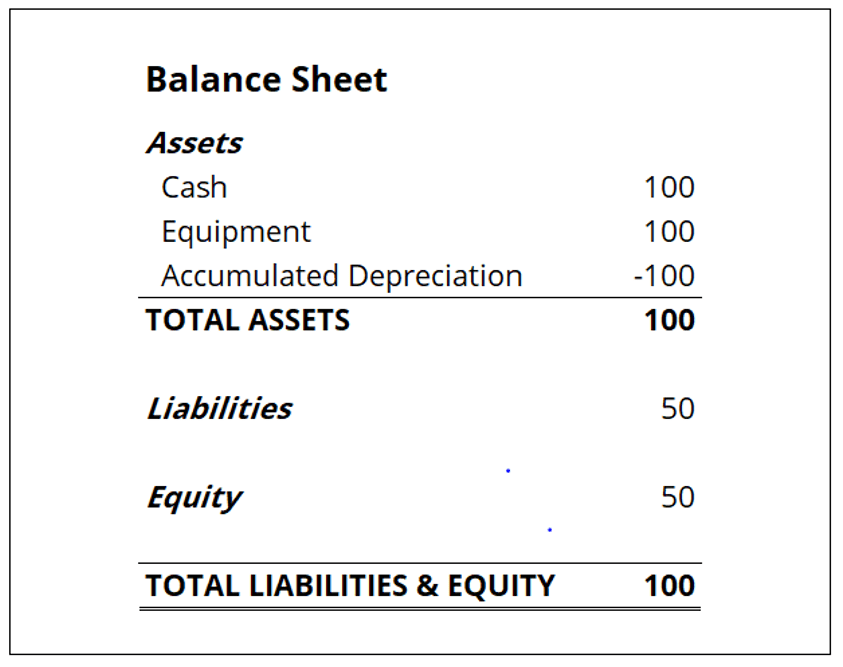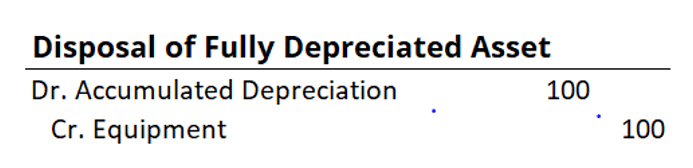# Fully Depreciated Asset

An asset whose worth is equivalent to its salvage value only for accounting purposes

## What is a Fully Depreciated Asset?

A fully depreciated asset is an accounting term used to describe an asset that is worth the same as its salvage value. An asset can become fully depreciated in two ways:

1. The asset expires its useful life
2. There is an impairment charge equal to or greater than the asset’s remaining value

If the asset’s accumulated depreciation is equivalent to the asset’s original cost, then it is classified as fully depreciated. If an impairment charge equal to the asset’s cost is incurred, then the asset is immediately fully depreciated.The depreciation expense accounting element does not fully reflect the actual used value of the equipment. It is more of an approximation that gives an estimate of the actual value used. For this reason, there are different methods to estimate the depreciation expense. When using more conservative accounting practices, it is typical to impose a more aggressive depreciation schedule and recognize expenses earlier. Sometimes, a fully depreciated asset can still provide value to a company. In such a case, the operating profits of a company will increase because no depreciation expenses will be recognized.

Whenever the asset is no longer used by a company or is sold, the asset is removed from the company’s balance sheet.

### Accounting for fully depreciated assets

Since property, plant, and equipment (PP&E) and accumulated depreciation are balance sheet items, the full depreciation of an asset will affect the company’s balance sheet. At the same time, the income statement is impacted because that is where the depreciation expense is recorded. There are two cases for accounting reporting for fully depreciated assets: the fully depreciated asset is still in production use or it is disposed of.

If the asset is still used in the company’s operations, the asset’s account and accumulated depreciation will still be reported on the company’s balance sheet. The reported asset’s value and accumulated depreciation will be equal, but no entry will be required until the asset is disposed of. On the income statement, the operating profit is likely to increase because the depreciation expense will no longer be recorded on the income statement.If the fully depreciated asset is disposed of, the asset’s value and accumulated depreciated will be written off from the balance sheet. In such a scenario, the effect on the income statement will be the same as if no depreciation expense happened. The accounting treatment for the disposal of a completely depreciated asset is a debit to the account for the accumulated depreciation and a credit for the asset account.CFI is the official provider of the Financial Modeling & Valuation Analyst (FMVA)™ certification program and other corporate finance training online. To advance your career, check out the additional CFI resources below:

• Accelerated Depreciation
• Depreciation Methods
• Depreciation Schedule
• Projecting Balance Sheet Items